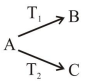# A radioactive nucleus decays by two different processes.

Question:

A radioactive nucleus decays by two different processes. The half life for the first process is $10 \mathrm{~s}$ and that for the second is $100 \mathrm{~s}$. the effective half life of the nucleus is close to:

1. $9 \mathrm{sec}$

2. $55 \mathrm{sec}$

3. $6 \mathrm{sec}$

4. $12 \mathrm{sec}$

Correct Option: 1,

Solution:$\frac{1}{\mathrm{~T}_{\text {eff }}}=\frac{1}{\mathrm{~T}_{1}}+\frac{1}{\mathrm{~T}_{2}}$

$\mathrm{T}_{\mathrm{eff}}=\frac{\mathrm{T}_{1} \mathrm{~T}_{2}}{\mathrm{~T}_{1}+\mathrm{T}_{2}}=\frac{1000}{110}=\frac{100}{11}=9.09$

$\mathrm{T}_{\mathrm{eff}} \cong 9$